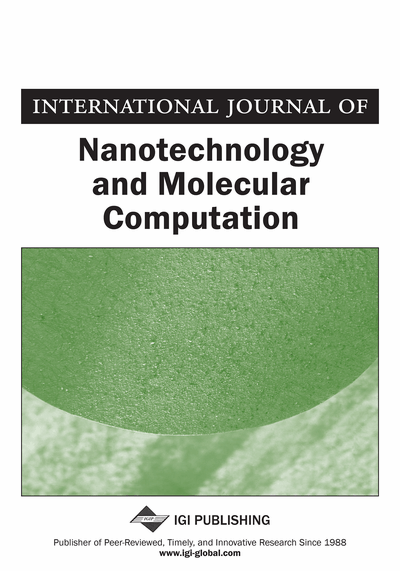# Algorithmic Models of Biochemical Dynamics: MP Grammars Synthetizing Complex Oscillators

Vincenzo Manca
DOI: 10.4018/ijnmc.2011100102
OnDemand:
(Individual Articles)
Available
\$29.50
No Current Special Offers

## Abstract

The paper presents the main ideas about MP grammars, a discrete formalism for describing and deducing dynamical systems, that combines ideas from formal language theory (P systems), recurrent equations, linear algebra and statistical regression. In this framework, some synthetic oscillators are presented which illustrate, in a simple manner, the expressive power of MP grammars in the analysis of interesting phenomena that are crucial in biochemical and biological contexts.
Article Preview
Top

## 1. Introduction

The theory of MP grammars (Metabolic P grammars) started in first years of 2000’s as a discrete mathematical method, inspired from P systems (an unconventional computation model based on abstract membranes (Păun, 2000; Gheorghe, 2005; Condon, Harel, Kok, Salomaa & Winfree, 2009; Păun, Rozenberg & Salomaa, 2010), for describing biological dynamics. A graphical, very useful, representation of these grammars in terms of MP graphs was defined in (Manca & Bianco, 2008). It turns out that MP grammars easily identify systems of first order Finite Difference (recurrent) Equations (FED). In the following years, algorithms and software were developed for simulating and reconstructing many biological phenomena (Bianco, Manca, Marchetti & Petterlini, 2007; Manca & Marchetti, 2012; Marchetti & Manca, 2012; Manca & Marchetti, 2013; Manca, Marchetti & Pagliarini, 2013; Castellini, Zucchelli, Busato & Manca, 2013). However, the main problem addressed in the MP theory, and systematically solved in many significant cases, is the so called Dynamical Inverse Problem (Manca & Marchetti, 2012, 2013; Manca, 2013), a very old problem in science (it was the starting problem of differential models of planetary orbits).

Let us recall that a dynamical system is given by a set of real variables changing in time and a set of “invariants”, that is, conditions (constraints) which are satisfied by the variables during their change. Let us observe the variables of a (discrete) dynamical system along a number of (equally spaced) time points (steps). The sequences of these values constitute a set of time series representing the behavior of the observed system. We pose the following question: can we reconstruct these time series as generated by certain kinds of interactions/transformations among the variables of the system? If this reconstruction is possible (even with some approximations), then we are able to infer an internal logic that is responsible of what we observe. Therefore, we can deduce a mechanism ruling the observed phenomenon, by passing from the time manifestation of the system to its state causation law (from the phenomenon to the noumenon, see Figure 1).

Oscillators are basic blocks of biological dynamics. In fact life rhythms are based on complex and correlated systems of oscillations keeping the basic physical and biochemical parameters within the correct ranges for maintaining the structures and mechanisms of life (Lotka, 1920; Volterra, 1926; Goldbeter, 1991, 1996, 2002).

In Manca, Franco and Scollo (2005) we discussed general dynamical concepts in a simple discrete framework of transition systems, and explained the computational relevance of periodicity. Here, we consider a notion of oscillation that extends the notion of quasi-periodicity considered in Manca, Franco and Scollo (2005), and also extends a previous approach to oscillations developed in Bianco and Manca (2006).

## Complete Article List

Search this Journal:
Reset
Volume 3: 4 Issues (2011)
Volume 2: 4 Issues (2010)
Volume 1: 4 Issues (2009)
View Complete Journal Contents Listing